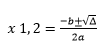код Quadratic Equation Solver - Calculatorology

 Enter a: Enter b: Enter c: The quadratic equation: x2 + x + = 0 Discriminat: Δ  = Quadratic formula: x1,2= First root: x1   = Second root: x2 =

The Quadratic equation solver is the simplest way of calculating a quadratic equation. You only need to determine the coefficients before entering them in the blank text fields. The full quadratic equation is ax2 + bx + c = 0, so you will enter the values of coefficients a, b and c respectively in their appropriate cells. Afterwards, click the ‘Calculate’ button to execute the conversion. The results will be displayed below the two controls indicating the discriminat, quadratic formula, first root and the second root. If you wish to calculate the new values, you will use the ‘Reset’ button to erase all the previous calculations at once from the text fields.

##### For example;
Find the first root and the second root of a quadratic equation, 4x2 + 2x + 1.

##### Solution
You will first enter the coefficients 4, 2 and 1 respectively as a, b and c. Click the calculate button to initiate the conversion. Your answers will be displayed as;

Discriminat Δ = -12

Quadratic formula X1, 2 = (-2 ± √ (-12)) / (2×4)

First root x1 = -0.25 + i0.43301270189

Second root X2 = -0.25 - i0.43301270189

The calculator uses particular formulas in performing the calculations. It makes it easier to solve the quadratic equations as it gives you the results within a single click.

Ax2 + bx + c = 0